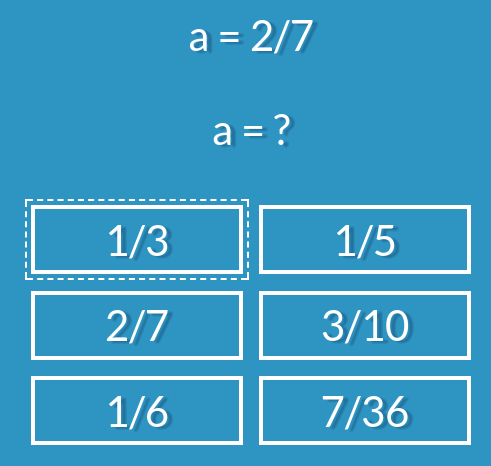Skip to content
{{ message }}

# h5p / h5p-arithmetic-quiz

Have a question about this project? Sign up for a free GitHub account to open an issue and contact its maintainers and the community.

By clicking “Sign up for GitHub”, you agree to our terms of service and privacy statement. We’ll occasionally send you account related emails.

Already on GitHub? Sign in to your account

# Merged linear equations into arithmetic quiz (HFP-1509) #8

Merged
merged 13 commits into from May 2, 2019
Merged

# Merged linear equations into arithmetic quiz (HFP-1509)#8

merged 13 commits into from May 2, 2019

## Conversation

###foxfabi commented Nov 11, 2017 • edited

 As discussed with @timothyylim i merged the linear equation H5P content type into arithmetic quiz. i tested the functionality in my drupal development environment. I hope the implementation meets your standard.
added 4 commits Nov 11, 2017
``` Merged Linear Equation Quiz ```
``` 079ba1c ```
`Merged Linear Equation Quiz into Arithmetic Quiz`
``` Merged Linear Equation Quiz ```
``` 9b48593 ```
`Merged Linear Equation Quiz into Arithmetic Quiz`
``` List of Sample equations ```
``` f8dcf97 ```
`Markdown of sample equations list`
``` Fixed question text for equations ```
``` 8ec7163 ```
```fixed the question visualization (2x = 9 = ?) to
2x = 9
x = ?```changed the title Merged linear equations into arithmetic quiz Merged linear equations into arithmetic quiz (HFP-1509) Nov 12, 2017
``` Fixed issues (HFP-1735/HFP-1509) ```
``` dbdd5c2 ```
```Fixed issues from HFP-1735: Added Quiz Type dropdown, Difficulty
dropdown with examples, Added fractions mode
Fixed issues from HFP-1509: Improved accessibility, Max value used in
equations operations is now hidden (set internally to 7)```

###timothyylim commented Nov 17, 2017

 Nice work! I've created another issue for this to be reviewed: https://h5ptechnology.atlassian.net/browse/HFP-1754
reviewed

###fnoks left a comment

 Great Work. I have left a few comments in the code. We should probably change the name of this content type to something like "Arithmetic and linear equation quiz"
 @@ -22,7 +22,10 @@ H5P.ArithmeticQuiz = (function (\$) { // Extend defaults with provided options self.options = \$.extend(true, {}, { intro: '', quizType: undefined,

#### fnoks Feb 13, 2018 Member

This should be set to the same value as the default in semantics.json (i.e. "arithmetic"). Otherwise, all existing content out there will break.

 .replaceAll('/', self.translations.divisionOperator) .replaceAll('÷', self.translations.divisionOperator) .replaceAll('=', self.translations.equalitySign); }

#### fnoks Feb 13, 2018 Member

Missing semicolon

 function randomOperation(operations, expr, useFractions) { // get a random operation var operation = operations[Math.floor(Math.random() * operations.length)]; number = randomNum(1, 7);

#### fnoks Feb 13, 2018 Member

"number" is not defined

 var equation = undefined; var solution = undefined; var number1 = undefined; var number2 = undefined;

#### fnoks Feb 13, 2018 Member

"number2" is assigned a value but never used

 // Generate 5 wrong ones: while (question.alternatives.length !== 5) { equation = generateEquation(question.variable, question.type, equationType, useFractions); var alternative = equation.toString();

#### fnoks Feb 13, 2018 Member

"alternative" is assigned but never used

 slideOfTotal: 'Slide :num of :total' } }, options); self.currentWidth = 0; self.gamePage = new H5P.ArithmeticQuiz.GamePage(self.options.arithmeticType, self.options.maxQuestions, self.options.UI, id); // Reset equation type if not selected if (self.options.quizType === H5P.ArithmeticQuiz.QuizType.ARITHMETIC) {

#### fnoks Feb 13, 2018 Member

I think this is unnecessary. Instead of checking arithmeticType/equationType, quizType should be used to indicate which mode we're in. That will make the code more readable.

 if (self.options.quizType === H5P.ArithmeticQuiz.QuizType.ARITHMETIC) { self.options.equationType = undefined; } self.gamePage = new H5P.ArithmeticQuiz.GamePage(self.options.arithmeticType, self.options.equationType, self.options.maxQuestions, self.options.useFractions, self.options.UI, id);

#### fnoks Feb 13, 2018 Member

Since this pull request is adding a new mode, I suggest the following:

• Create a separate question generator class per mode (that offers the same public "interface"/functions).
• Initialize the correct generator here, and dependency inject the instance into GamePage

Please let me know if this is unclear :)

###fnoks commented Feb 13, 2018

 I also wonder if the generator should create tasks like this:added 8 commits Feb 14, 2018
``` This should be set to the same value as the default in semantics.json… ```
``` 71b8205 ```
`… (i.e. "arithmetic"). Otherwise, all existing content out there will break.`
``` Missing semicolon ```
``` a74baa6 ```
``` "number" is not defined ```
``` 8c1046f ```
``` "number2" is assigned a value but never used ```
``` 9eb7c96 ```
``` "alternative" was assigned but never used ```
``` da2fedc ```
``` Refactoring Question generator ```
``` b1abcae ```
``` Refactoring Question generator ```
``` 441ea4f ```
``` Some of the alternatives get wider than the box their in ```
``` b6f5fea ```
`Fixed generator that should not create tasks like this: a = 2/7`

###fnoks commented May 23, 2018 • edited

 I have reopened the issue, so the new changes will be reviewed! https://h5ptechnology.atlassian.net/browse/HFP-1509
to join this conversation on GitHub. Already have an account? Sign in to comment
Labels
None yet
Projects
None yet
Linked issues

Successfully merging this pull request may close these issues.

None yet